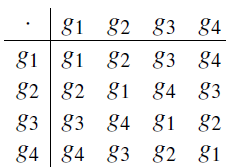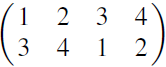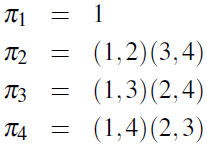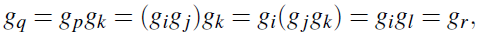Symmetric groups and Cayley’s Theorem: Cayley’s Theorem is one of the reasons why the symmetric groups form such an important class of groups: in a sense, if we understand the symmetric groups completely, then we understand all groups!

Theorem 3.29 Every group is isomorphic to a subgroup of some symmetric group. Before we give the proof, here is a small digression on the background. Group theory began in the late 18th century: Lagrange, for example, proved his theorem in 1770. Probably the first person to write down the axioms for a group in anything like the present form was Dyck in 1882. So what exactly were group theorists doing for a hundred years?
The answer is that Lagrange, Galois, etc. regarded a group as a set G of permutations with the properties
• G is closed under composition;
• G contains the identity permutation;
• G contains the inverse of each of its elements.
In other words, G is a subgroup of the symmetric group.

Thus, Cayley’s contribution was to show that every group (in the modern sense) could be regarded as a group of permutations; that is, every structure which satisfies the group axioms can indeed be thought of as a group in the sense that Lagrange and others would have understood.
In general, systems of axioms in mathematics are usually not invented out of the blue, but are an attempt to capture some theory which already exists.
Proof of Cayley’s Theorem: We begin with an example. Here is the Cayley table of a group we have met earlier: it is C2 ×C2, or the additive group of the field of four elements. its elements were called e,a,b,c; now I will call them g1,g2,g3,g4.Now consider the four columns of this table. In each column, we see the four group elements g1, . . . ,g4, each occurring once; so their subscripts form a permutation of {1,2,3,4}. Let πi be the permutation which is given by the ith column. For example, for i = 3, the elements of the column are (g3,g4,g1,g2), and so p3 is the permutation which isin two-line notation, or (1,3)(2,4) in cycle notation.
The four permutations which arise in this way are:Now we claim that {π1,π2,π3,π4} is a subgroup H of the symmetric group S4, and that the map θ defined by giθ = πi is an isomorphism from G to H. (This means that, if gigj = gk, then πiπj = πk, where permutations are composed in the usual way.) This can be verified with a small amount of checking.

You might think that it would be easier to use rows instead of columns in this argument. In the case of an Abelian group, like the one in this example, of course it makes no difference since the Cayley table is symmetric; but for non-Abelian groups, the statement would not be correct for rows. So now we come to a more precise statement of Cayley’s Theorem. We assume here that the group is finite; but the argument works just as well for infinite groups too.

Theorem 3.30 (Cayley’s Theorem) Let G = {g1, . . . ,gn} be a finite group. For j ∈ {1, . . . ,n}, let pj be the function from {1, . . . ,n} to itself defined by the rule

ipj = k if and only if gigj = gk. Then
(a) pj is a permutation of {1, . . . ,n};
(b) the set H = {π1, . . . ,πn} is a subgroup of Sn;
(c) the map q : G!Sn given by gjq = πj is a homomorphism with kernel {1} and image H;
(d) G is isomorphic to H.
Proof (a) To show that pj is a permutation, it is enough to show that it is oneto- one, since a one-to-one function on a finite set is onto. (For infinite groups,
we would also have to prove that it is onto.) So suppose that i1πj = i2πj = k. This means, by definition, that gi1gj = gi2gj = gk. Then by the cancellation law,
gi1 = gi2 = gkg−1 j , and so i1 = i2.
(c) Clearly the image of q is H. So if we can show that q is a homomorphism, the fact that H is a subgroup of Sn will follow.

Suppose that gjgk = gl . We have to show that pjpk = πl , in other words (since these are functions) that (iπpj)πk =iπl for any i 2 {1, . . . ,n}. Define numbers p,θ, r
by gigj = gπ, gπgk = gq, and gigl = gr. Then iπj = π, ππk = θ (so iπjπk = θ), and iπl = r. So we have to prove that θ = r. ButNow the kernel of θ is the set of elements gj for which pj is the identity permutation. Suppose that gj 2 Ker(q). Then iπj = i for any i 2 {1, . . . ,n}. This means that gigj = gi, so (by the cancellation law) gj is the identity. So Ker(θ) = {1}.

Now the First Isomorphism Theorem shows that H = Im(q) is a subgroup of Sn (that is, (b) holds), and that
G = G/{1} = G/Ker(θ) = H,
that is, (d) holds. So we are done.